# 支持向量机分类实战

+关注继续查看

SVM主要的思想可以概括为两点：

1ï¼ 它是针对线性可分情况进行分析，对于线性不可分的情况，通过使用非线性映射算法将低维输入空间线性不可分的样本转化为高维特征空间使其线性可分，从而使得高维特征空间采用线性算法对样本的非线性特征进行线性分析成为可能。

2ï¼ 它基于结构风险最小化理论之上在特征空间中构建最优超平面，使得学习器得到全局最优化，并且在整个样本空间的期望以某种概率满足一定上界。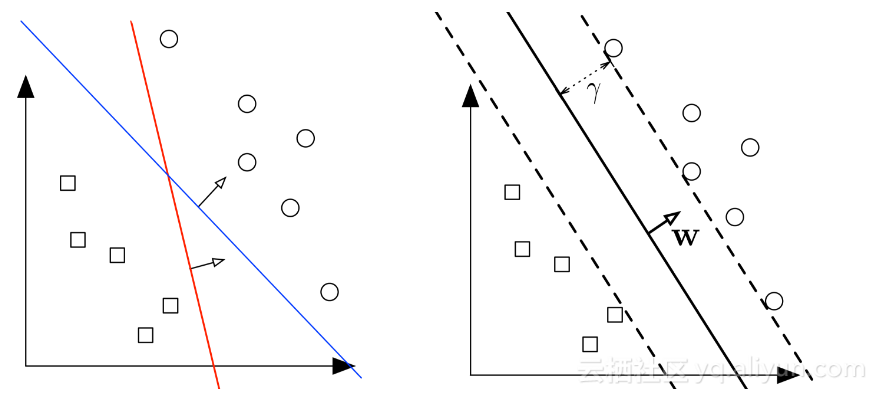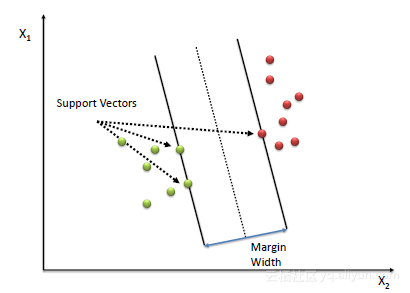1）只有数据线性分离才有效。

2）对异常值非常敏感。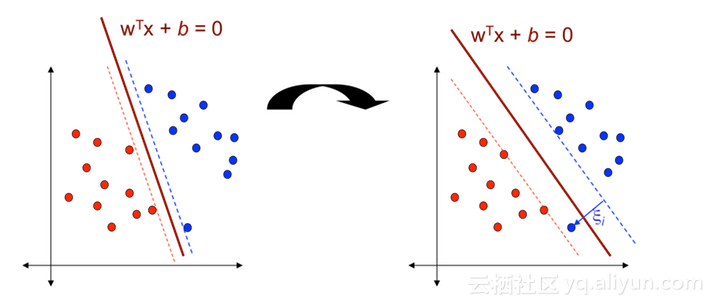## 非线性SVM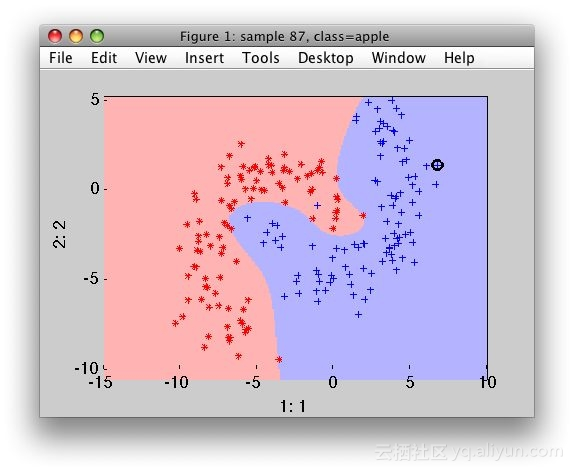## 核心技巧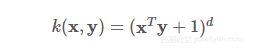xy）= eγx - y |2γ0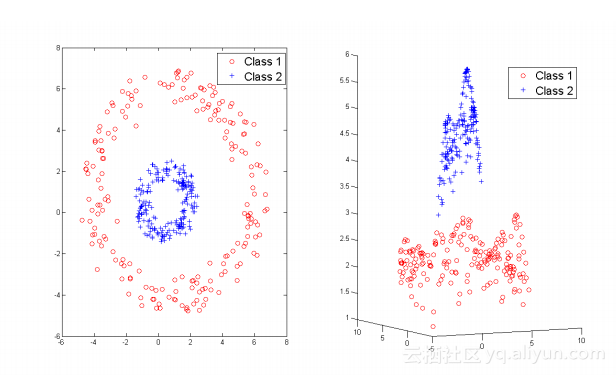1.C参数

C参数决定SVM分类器的边距宽度，而且它的分类器严格，因此边缘宽度较小。对于值大的C，如果该超平面更好地将所有训练点归类正确，则该模型将选择较小余量的超平面。相反，C的非常小的值将导致模型寻找更大的边缘从而分离超平面。对于非常小的C值，你应该得到错误分类的例子，通常即使你的训练数据是线性分离的。

2.γ参数

## 应用scikit实现SVM

import numpy as np
import pandas as pd
from matplotlib import style
from sklearn.svm import SVC
import matplotlib.pyplot as plt
plt.rcParams['figure.figsize'] = (12, 6)
style.use('ggplot')
# Import Dataset
X = data.values[:, :2]
y = data.values[:, 2]
# A function to draw hyperplane and the margin of SVM classifier
def draw_svm(X, y, C=1.0):
# Plotting the Points
plt.scatter(X[:,0], X[:,1], c=y)
# The SVM Model with given C parameter
clf = SVC(kernel='linear', C=C)
clf_fit = clf.fit(X, y)
# Limit of the axes
ax = plt.gca()
xlim = ax.get_xlim()
ylim = ax.get_ylim()
# Creating the meshgrid
xx = np.linspace(xlim, xlim, 200)
yy = np.linspace(ylim, ylim, 200)
YY, XX = np.meshgrid(yy, xx)
xy = np.vstack([XX.ravel(), YY.ravel()]).T
Z = clf.decision_function(xy).reshape(XX.shape)
# Plotting the boundary
ax.contour(XX, YY, Z, colors='k', levels=[-1, 0, 1],
alpha=0.5, linestyles=['--', '-', '--'])
ax.scatter(clf.support_vectors_[:, 0],
clf.support_vectors_[:, 1],
s=100, linewidth=1, facecolors='none')
plt.show()
# Returns the classifier
return clf_fit
clf_arr = []
clf_arr.append(draw_svm(X, y, 0.0001))
clf_arr.append(draw_svm(X, y, 0.001))
clf_arr.append(draw_svm(X, y, 1))
clf_arr.append(draw_svm(X, y, 10))
for i, clf in enumerate(clf_arr):
# Accuracy Score
print(clf.score(X, y))
pred = clf.predict([(12, 32), (-250, 32), (120, 43)])
print(pred)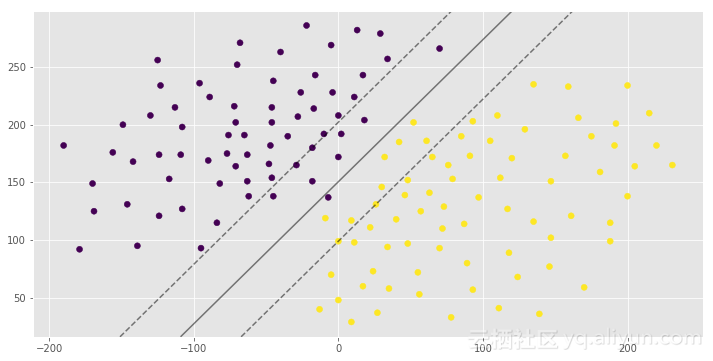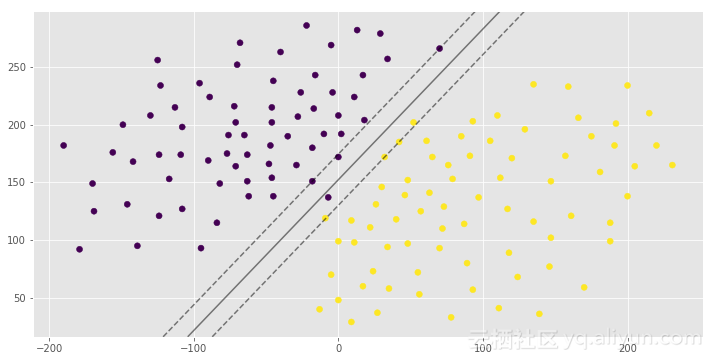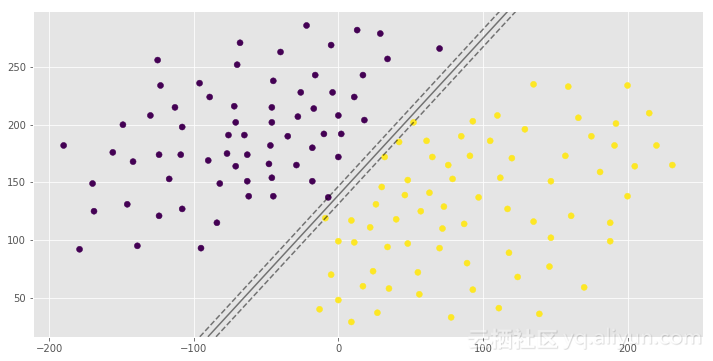0.992907801418
[1 0 1]
0.992907801418
[1 0 1]
1.0
[1 0 1]
1.0

[1 0 1]

import numpy as np
import pandas as pd
from matplotlib import style
from sklearn.svm import SVC
import matplotlib.pyplot as plt
plt.rcParams['figure.figsize'] = (12, 6)
style.use('ggplot')
X = data.values[:, :2]
y = data.values[:, 2]
def draw_svm(X, y, C=1.0):
plt.scatter(X[:,0], X[:,1], c=y)
clf = SVC(kernel='poly', C=C)
clf_fit = clf.fit(X, y)
ax = plt.gca()
xlim = ax.get_xlim()
ylim = ax.get_ylim()
xx = np.linspace(xlim, xlim, 200)
yy = np.linspace(ylim, ylim, 200)
YY, XX = np.meshgrid(yy, xx)
xy = np.vstack([XX.ravel(), YY.ravel()]).T
Z = clf.decision_function(xy).reshape(XX.shape)
ax.contour(XX, YY, Z, colors='k', levels=[-1, 0, 1],
alpha=0.5, linestyles=['--', '-', '--'])
ax.scatter(clf.support_vectors_[:, 0],
clf.support_vectors_[:, 1],
s=100, linewidth=1, facecolors='none')
plt.show()
return clf_fit
clf = draw_svm(X, y)
score = clf.score(X, y)
pred = clf.predict([(-130, 110), (-170, -160), (80, 90), (-280, 20)])
print(score)
print(pred)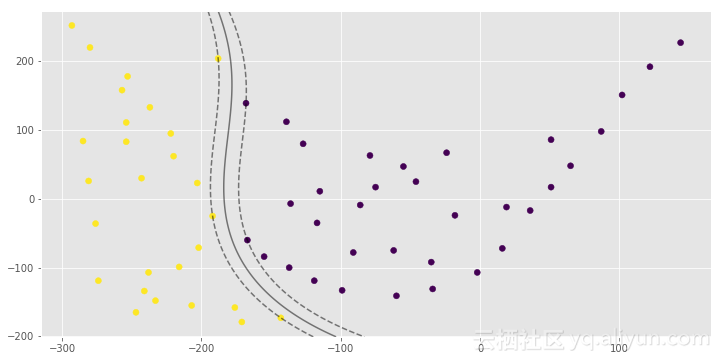1.0
[0 1 0 1]

import numpy as np
import pandas as pd
from matplotlib import style
from sklearn.svm import SVC
from sklearn.datasets import make_classification, make_blobs, make_moons
import matplotlib.pyplot as plt
plt.rcParams['figure.figsize'] = (12, 6)
style.use('ggplot')
X, y = make_moons(n_samples=200)
# Auto gamma equals 1/n_features
def draw_svm(X, y, C=1.0, gamma='auto'):
plt.scatter(X[:,0], X[:,1], c=y)
clf = SVC(kernel='rbf', C=C, gamma=gamma)
clf_fit = clf.fit(X, y)
ax = plt.gca()
xlim = ax.get_xlim()
ylim = ax.get_ylim()
xx = np.linspace(xlim, xlim, 200)
yy = np.linspace(ylim, ylim, 200)
YY, XX = np.meshgrid(yy, xx)
xy = np.vstack([XX.ravel(), YY.ravel()]).T
Z = clf.decision_function(xy).reshape(XX.shape)
ax.contour(XX, YY, Z, colors='k', levels=[-1, 0, 1],
alpha=0.5, linestyles=['--', '-', '--'])
ax.scatter(clf.support_vectors_[:, 0],
clf.support_vectors_[:, 1],
s=100, linewidth=1, facecolors='none')
plt.show()
return clf_fit
clf_arr = []
clf_arr.append(draw_svm(X, y, 0.01))
clf_arr.append(draw_svm(X, y, 0.1))
clf_arr.append(draw_svm(X, y, 1))
clf_arr.append(draw_svm(X, y, 10))
for i, clf in enumerate(clf_arr):
print(clf.score(X, y))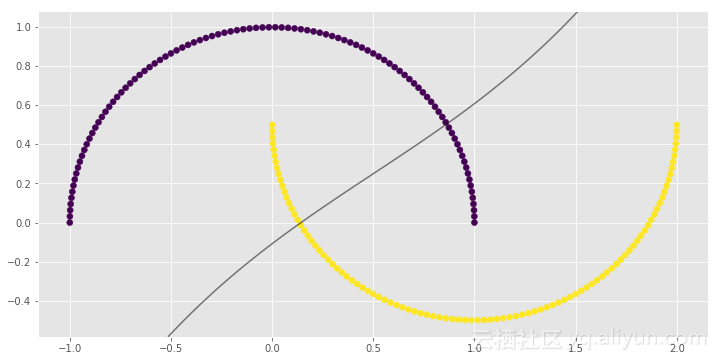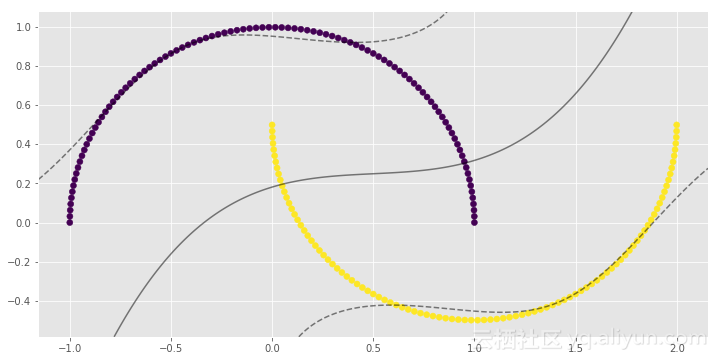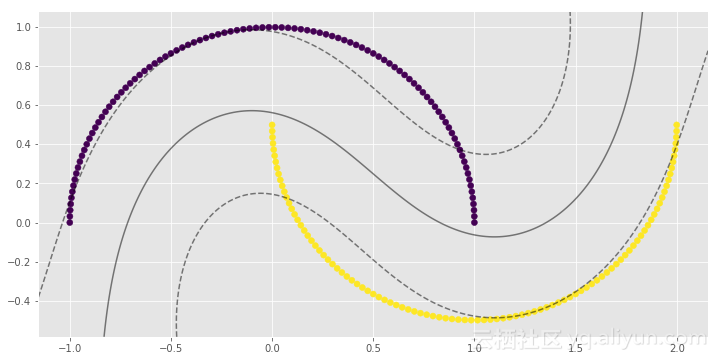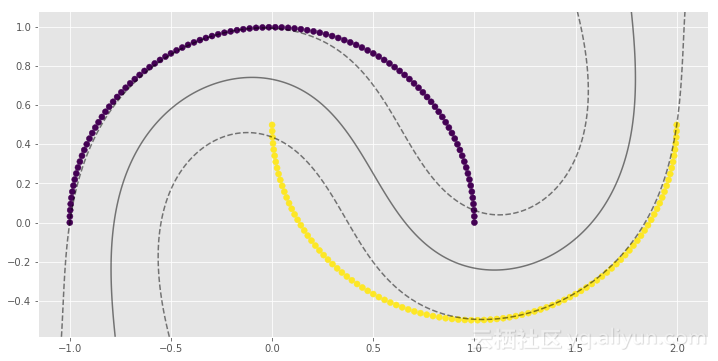0.83

0.9
1.0
1.0

import numpy as np
import pandas as pd
from matplotlib import style
from sklearn.svm import SVC
from sklearn.datasets import make_gaussian_quantiles
import matplotlib.pyplot as plt
plt.rcParams['figure.figsize'] = (12, 6)
style.use('ggplot')
X, y = make_gaussian_quantiles(n_samples=200, n_features=2, n_classes=2, cov=3)
# Auto gamma equals 1/n_features
def draw_svm(X, y, C=1.0, gamma='auto'):
plt.scatter(X[:,0], X[:,1], c=y)
clf = SVC(kernel='rbf', C=C, gamma=gamma)
clf_fit = clf.fit(X, y)
ax = plt.gca()
xlim = ax.get_xlim()
ylim = ax.get_ylim()
xx = np.linspace(xlim, xlim, 200)
yy = np.linspace(ylim, ylim, 200)
YY, XX = np.meshgrid(yy, xx)
xy = np.vstack([XX.ravel(), YY.ravel()]).T
Z = clf.decision_function(xy).reshape(XX.shape)
ax.contour(XX, YY, Z, colors='k', levels=[-1, 0, 1],
alpha=0.5, linestyles=['--', '-', '--'])
ax.scatter(clf.support_vectors_[:, 0],
clf.support_vectors_[:, 1],
s=100, linewidth=1, facecolors='none')
plt.show()
return clf_fit
clf_arr = []
clf_arr.append(draw_svm(X, y, 0.1))
clf_arr.append(draw_svm(X, y, 1))
clf_arr.append(draw_svm(X, y, 10))
clf_arr.append(draw_svm(X, y, 100))
for i, clf in enumerate(clf_arr):
print(clf.score(X, y))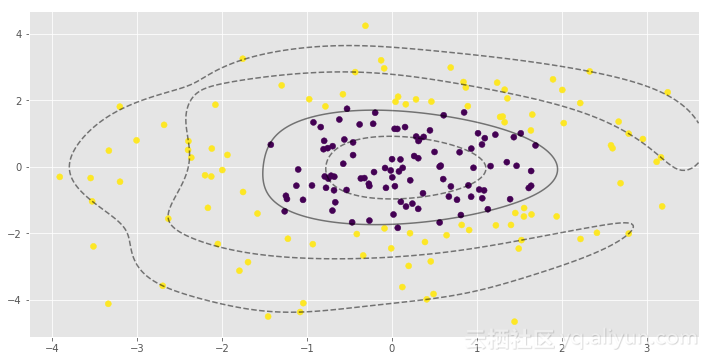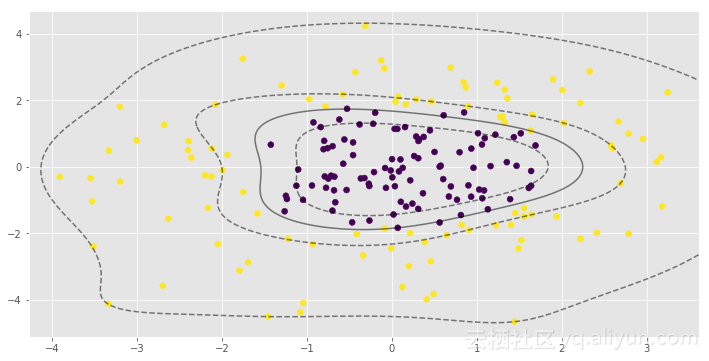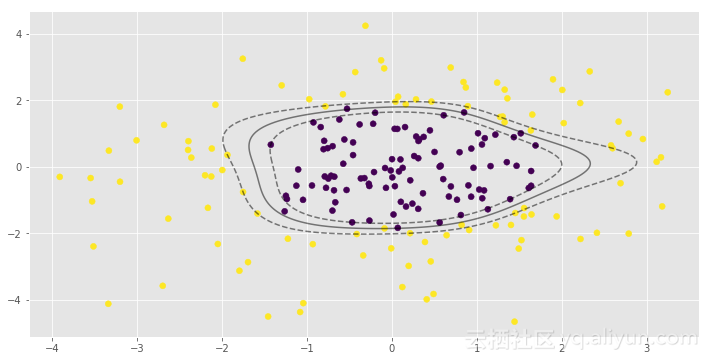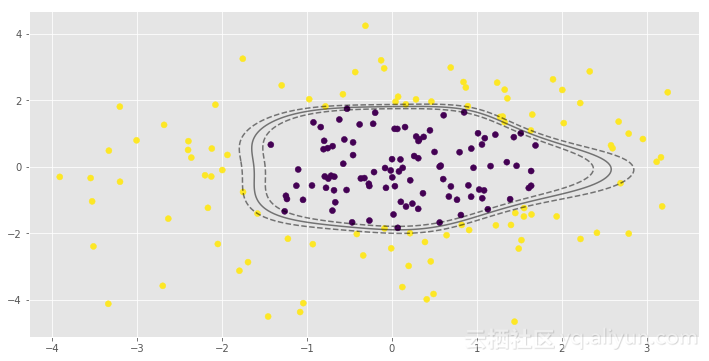0.965
0.97
0.985
0.995

γ参数对RBF SVM模型非常重要。在第一个例子中，γ低值导致非线性分类几乎接近线性分类。|
6月前
|

114 0
|
7月前
|

81 0
|
9月前
|

【SVM分类】基于最小二乘支持向量机实现数据分类附matlab代码
【SVM分类】基于最小二乘支持向量机实现数据分类附matlab代码
125 0
|

|
Python

840 0